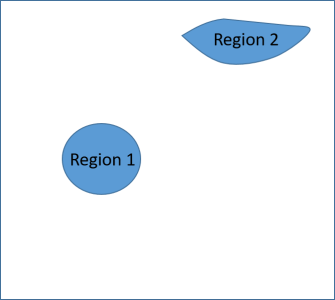# Normalization of different regions in imageHi all,

How can I divide different regions of image by specific value? In the attached image, you can see two selected regions of interest. I need to divide all intensities in Region 1 by value A, and divide all intensities in Region 2 by value B. Is it possible?I define region using Image --> Image ROI --> Start ROI editing, select ROI, finish ROI editing.

But if I create 2D normalization matrix, how can I determine, which numbers in that matrix should I set equal to A, which pixels should I set equal to B, and which pixels should I set equal to 1?

I have never used that functionality before, but after having a quick look it looks like you can save your region of interest by clicking the Save ROI Copy button. That will create the wave M_ROIMask in root, in which all pixels in the region of interest is one and all pixels outside the region of interest is zero.

To use this to normalize two regions differently you have to create two mask waves: one for Region 1 and one for Region 2. Divide the Region 1 mask wave with the normalization factor needed for region 1 and divide the Region 2 mask wave with the normalization factor needed for Region 2. Now add the two waves together. This will create a wave with two different normalization factors for the two regions and zero everywhere else. Now multiply your image with this combined mask wave.

Thank you!

But when I divide each Region mask wave with corresponding normalization factors, I get zeros everywhere. And the final image is white at all. Since mask can only have two values, zero and one, when I divide, for example, by 10, I obtain zeros instead if 0.1 in selected region in mask wave.

olelytken wrote:

You can convert the mask wave into floating point using Redimension /D M_ROIMask. That should hopefully do the trick.

I converted mask waves into floating point, divided each mask wave by corresponding normalization factor, summed these two mask waves, and then muliplied by original image. It resulted in image with two regions with normalized intensities, but all pixels outside these regions have zero intensity. Is there any opportunity to preserve intensities for all pixels outside the regions of interest, i.e. to leave them the same as in the original image?

Physicist92 wrote:

It resulted in image with two regions with normalized intensities, but all pixels outside these regions have zero intensity. Is there any opportunity to preserve intensities for all pixels outside the regions of interest, i.e. to leave them the same as in the original image?

Set the zero value pixels in the mask to one using:

`mask[][] = mask[p][q] == 0 ? 1 : mask[p][q]`

Where mask is your mask wave.  Any zero value pixel is changed to one and any non-zero value pixel retains its value.

The help entry for this operator reads, in part:

? : is the conditional operator, which is a shorthand form of an if-else-endif statement. It is of the form:
<expression> ? <TRUE> : <FALSE>
When expression is nonzero, it evaluates the TRUE part, otherwise it evaluates the FALSE part.ForumSupportGallery